## Schwarzschild De Sitter Metric

English Version
Yukterez
Beiträge: 269
Registriert: Mi 21. Okt 2015, 02:16

### Schwarzschild De Sitter MetricThis is the english version.Deutschsprachige Version: hier entlangThe Schwarzschild De Sitter metric can be used to study the gravity of a dominant mass in an expanding universe:The critical mass at which the black hole horizon coincides with the cosmic event horizon is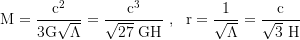where the radii of the horizons are derived by setting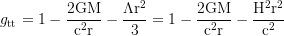to 0 and solving for the positive solutions for r.The maximal mass of a black hole in a De Sitter universe with our cosmological constant that is Λ≈1e-52/m² would be approximately 4.3e52kg:x-axis: M (in kg), y-axis: r (in GLyr); green/blue: horizons (cosmic event horizon and black hole horizon), red: Schwarzschild radius rsThe attraction of a galaxy like ours is offset by the expansion of the universe at a distance of about 1 megaparsec, so that a test particle that is at that distance from the galaxy is attracted by it as much as it is accelerated away from it by the dark energy.

The physical distance of a test particle from the dominant mass remains constant at the equilibrium radius (while the distance in co-moving coordinates is shrinking). If the dominant mass rests relative to the background radiation, i.e. flows with the Hubbleflow, our stationary test particle can no longer also rest relative to the background radiation, since it is not flowing with the Hubble flow when it is stationary with respect to the central mass.

Equilibrium radius: the neutonian orbital velocity is set equal to the vacuum recession velocity and resolved to r: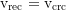Derivation: the equilibrium radius at which a test particle remains stationary relative to the dominant mass is, withand the resulting radial component of the four-accelerationwhich is set to 0 and resolved to r, atIn a De Sitter universe, the relation between the Hubble constant and the cosmological constant isplugging that into the solution for the equilibrium radius, we get the solution obtained aboveFor a circular orbit around the locally dominant mass we set

and solve for v:which is an angular velocity ofwhere for brevity we use the dimensionless length and cosmological constantThe local escape velocity relative to a stationary buoy is withor E=-pt=mc² and resolved to vwhere the minimum is always greater than 0 and lies at the equilibrium radius mentioned above, where it is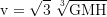For an observer who is traveling with the escape velocity, the gravitational and the kinematic time dilation balance out, so that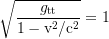The frames of those local observers build the hypersurface of constant t in comoving coordinates.Recessional velocity of free-falling test particles that arise near the equilibrium radius (in natural units of G=M=c=1 und Λ=0.01):x-axis: r (in GM/c²), y-axis: v (in c); red: recessional velocity relative to stationary Fidos, green: escape velocity. Λ=c⁴/G²/M²/100=1/rs²/25The worksheets (.nb format) can be downloaded by clicking on the respective image. Complete equations of motion: SSdS, Related topics: click here and here
/blank]Simon Tyran @ minds || gab || parler || wikipedia || stackexchange || wolfram